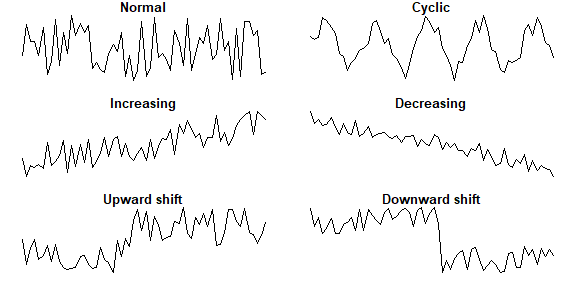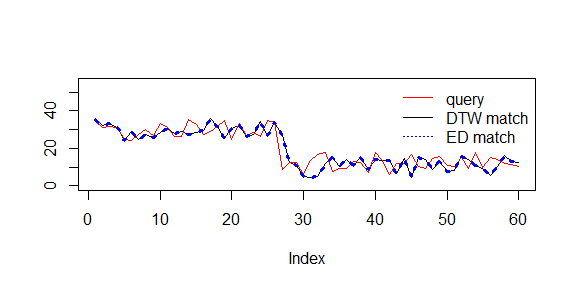# Using rucrdtw

## Introduction

Dynamic Time Warping (DTW) methods provide algorithms to optimally map a given time series onto all or part of another time series (Berndt and Clifford 1994). The remaining cumulative distance between the series after the alignment is a useful distance metric in time series data mining applications for tasks such as classification, clustering, and anomaly detection.

Calculating a DTW alignment is computationally relatively expensive, and as a consequence DTW is often a bottleneck in time series data mining applications. The UCR Suite (Rakthanmanon et al. 2012) provides a highly optimized algorithm for best-match subsequence searches that avoids unnecessary distance computations and thereby enables fast DTW and Euclidean Distance queries even in data sets containing trillions of observations.

A broad suite of DTW algorithms is implemented in R in the dtw package (Giorgino 2009). The rucrdtw R package provides complementary functionality for fast similarity searches by providing R bindings for the UCR Suite via Rcpp (Eddelbuettel and Francois 2011). In addition to queries and data stored in text files, rucrdtw also implements methods for queries and/or data that are held in memory as R objects, as well as a method to do fast similarity searches against reference libraries of time series.

## Installation

Install rucrdtw from GitHub:

install.packages("devtools")
devtools::install_github("pboesu/rucrdtw")

## Examples

Load rucrdtw package:

library("rucrdtw")

create a random long time series

set.seed(123)
rwalk <- cumsum(runif(1e7, min = -0.5, max = 0.5))

Pick a random subsequence of 100 elements as a query

qstart <- sample(length(rwalk), 1)
query <- rwalk[qstart:(qstart+100)]

Since both query and data are R vectors, we use the vector-vector methods for the search.

system.time(dtw_search <- ucrdtw_vv(data = rwalk, query = query, dtwwindow = 0.05))
##    user  system elapsed
##    1.61    0.00    1.62
all.equal(qstart, dtw_search$location) ##  TRUE system.time(ed_search <- ucred_vv(data = rwalk, query = query)) ## user system elapsed ## 1.12 0.00 1.12 all.equal(qstart, ed_search$location)
##  TRUE

And in a matter of seconds we have searched 10 million data points and rediscovered our query!

Searching for an exact match, however, is somewhat artificial. The real power of the similarity search is finding structurally similar subsequences in complex sets of time series. To demonstrate this we load an example data set:

data("synthetic_control")

This data set contains 600 time series of length 60 from 6 classes (Alcock et al. 1999). The data set documentation contains further information about these data. It can be displayed using the command ?synthetic_control. We can plot an example of each class

par(mfrow = c(3,2),
mar = c(1,1,1,1))
classes = c("Normal", "Cyclic", "Increasing", "Decreasing", "Upward shift", "Downward shift")
for (i in 1:6){
plot(synthetic_control[i*100-99,], type = "l", xaxt = "n", yaxt = "n", ylab="", xlab = "", bty="n", main=classes[i])
}Since we are now comparing a query against a set of time series, we only need to do comparisons for non-overlapping data sequences. The matrix-vector methods ucrdtw_mv and ucred_mv provide this functionality.

We can demonstrate this by removing a query from the data set, and then searching for a closest match:

index <- 600
query <- synthetic_control[index,]

dtw_search <- ucrdtw_mv(synthetic_control[-index,], query, 0.05, byrow = TRUE)
ed_search <- ucred_mv(synthetic_control[-index,], query, byrow= TRUE)

And plot the results:

plot(synthetic_control[dtw_search$location,], type="l", ylim=c(0,55), ylab="") lines(query, col="red") lines(synthetic_control[ed_search$location,], col="blue", lty=3, lwd=3)
legend("topright", legend = c("query", "DTW match", "ED match"), col=c("red", "black", "blue"), lty=c(1,1,3), bty="n")